# EECS 122 Introduction to Computer Networks Performance Modeling

• Slides: 32EECS 122: Introduction to Computer Networks Performance Modeling Computer Science Division Department of Electrical Engineering and Computer Sciences University of California, Berkeley, CA 94720 -1776 EECS 122 - UCB Katz, Stoica F 04Outline § § Motivations Timing diagrams Metrics Evaluation techniques Katz, Stoica F 04 2Motivations § § § § Understanding network behavior Improving protocols Verifying correctness of implementation Detecting faults Monitor service level agreements Choosing provides Billing Katz, Stoica F 04 3Outline § Ø § § Motivations Timing diagrams Metrics Evaluation techniques Katz, Stoica F 04 4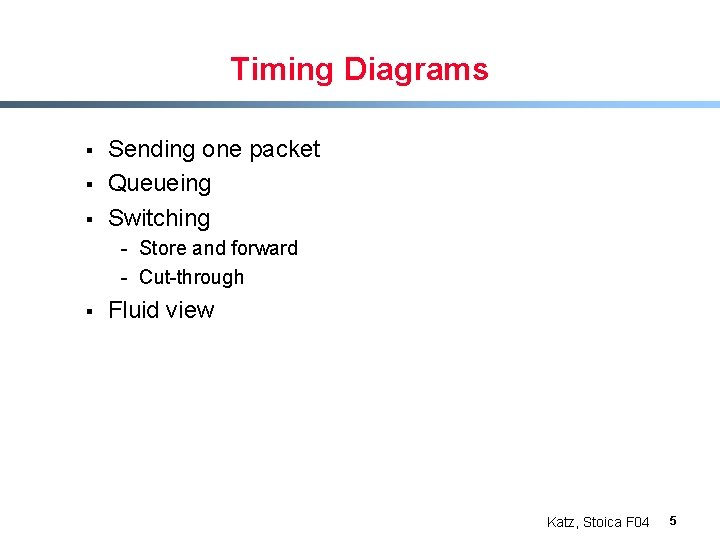Timing Diagrams § § § Sending one packet Queueing Switching - Store and forward - Cut-through § Fluid view Katz, Stoica F 04 5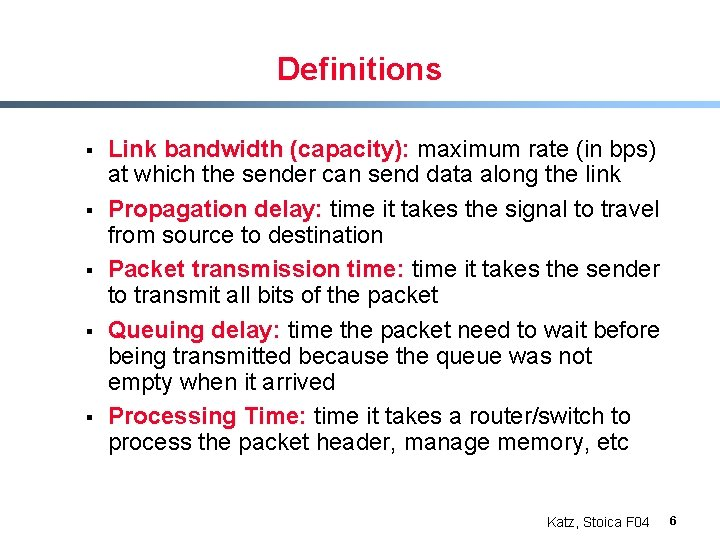Definitions § § § Link bandwidth (capacity): maximum rate (in bps) at which the sender can send data along the link Propagation delay: time it takes the signal to travel from source to destination Packet transmission time: time it takes the sender to transmit all bits of the packet Queuing delay: time the packet need to wait before being transmitted because the queue was not empty when it arrived Processing Time: time it takes a router/switch to process the packet header, manage memory, etc Katz, Stoica F 04 6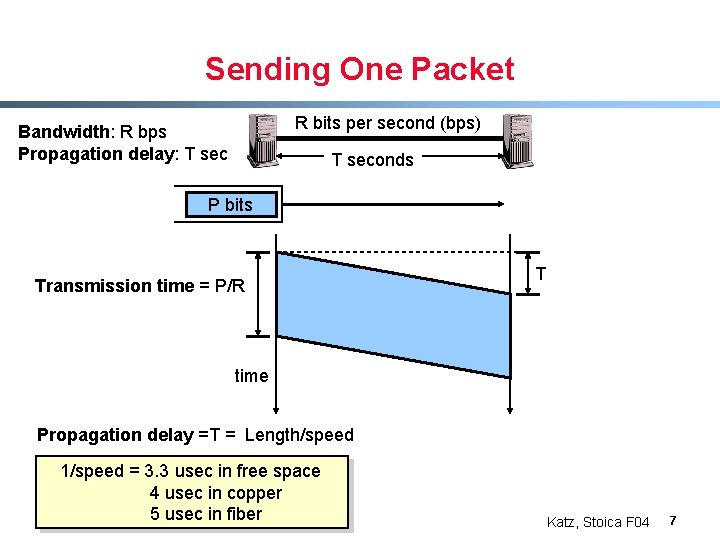Sending One Packet R bits per second (bps) Bandwidth: R bps Propagation delay: T seconds P bits Transmission time = P/R T time Propagation delay =T = Length/speed 1/speed = 3. 3 usec in free space 4 usec in copper 5 usec in fiber Katz, Stoica F 04 7Sending one Packet: Examples P = 1 Kbyte R = 1 Gbps 100 Km, fiber => T = 500 usec P/R = 8 usec T >> P/R T P/R time T << P/R T P = 1 Kbyte R = 100 Mbps 1 Km, fiber => T = 5 usec P/R = 80 usec P/R time Katz, Stoica F 04 8Queueing § The queue has Q bits when packet arrives packet has to wait for the queue to drain before being transmitted P bits Q bits Capacity = R bps Propagation delay = T sec Queueing delay = Q/R P/R T time Katz, Stoica F 04 9Queueing Example P bits Q bits P = 1 Kbit; R = 1 Mbps P/R = 1 ms Packet arrival Q(t) Time (ms) 0 0. 5 1 7 7. 5 Delay for packet that arrives at time t, d(t) = Q(t)/R + P/R 2 Kb 1. 5 Kb 1 Kb 0. 5 Kb Time packet 1, d(0) = 1 ms packet 2, d(0. 5) = 1. 5 ms packet 3, d(1) = 2 ms Katz, Stoica F 04 10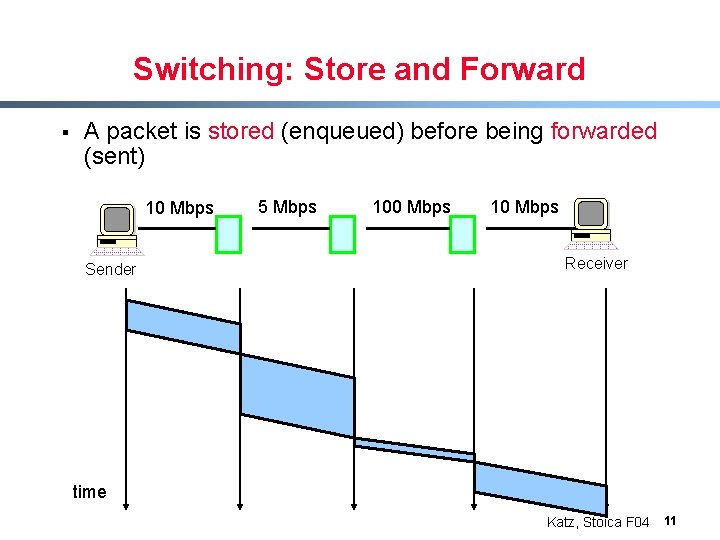Switching: Store and Forward § A packet is stored (enqueued) before being forwarded (sent) 10 Mbps Sender 5 Mbps 100 Mbps 10 Mbps Receiver time Katz, Stoica F 04 11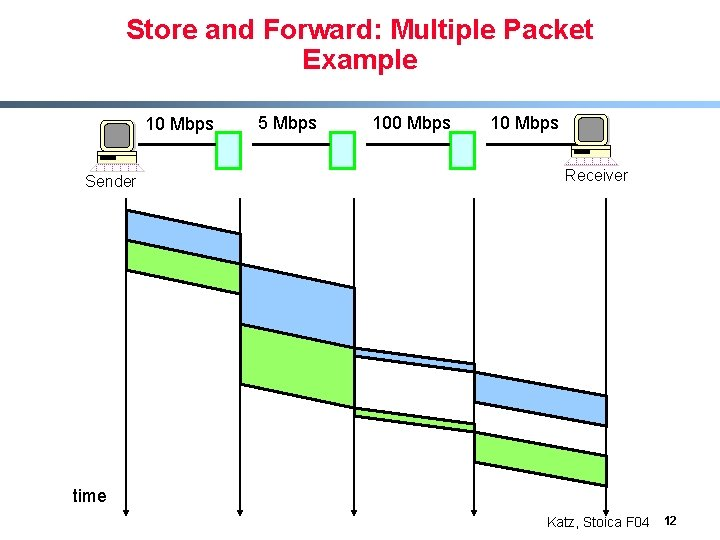Store and Forward: Multiple Packet Example 10 Mbps Sender 5 Mbps 100 Mbps 10 Mbps Receiver time Katz, Stoica F 04 12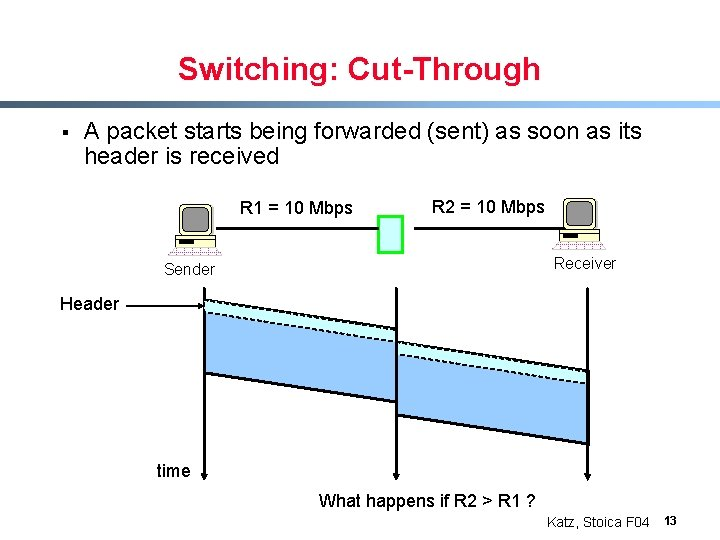Switching: Cut-Through § A packet starts being forwarded (sent) as soon as its header is received R 1 = 10 Mbps R 2 = 10 Mbps Receiver Sender Header time What happens if R 2 > R 1 ? Katz, Stoica F 04 13Fluid Flow System § Packets are serviced bit-by-bit as they arrive Q(t) = queueing size at time t a(t) – arrival rate Q(t) e(t) – departure rate Rate or Queue size a(t) e(t) Q(t) t Katz, Stoica F 04 14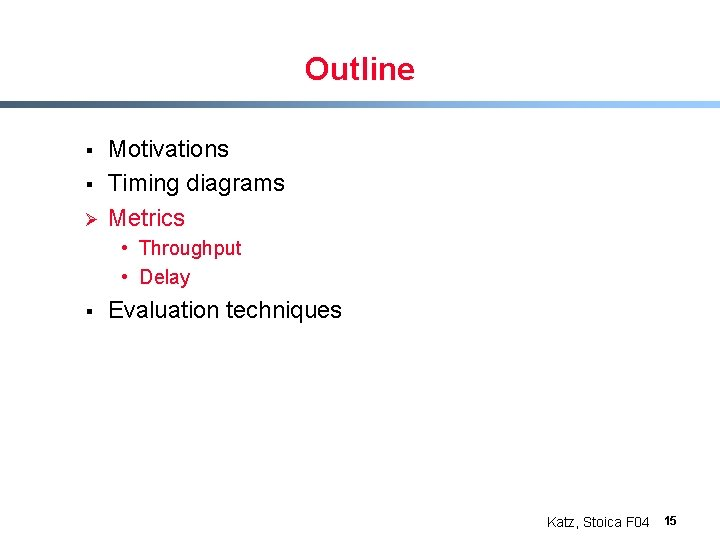Outline § § Ø Motivations Timing diagrams Metrics • Throughput • Delay § Evaluation techniques Katz, Stoica F 04 15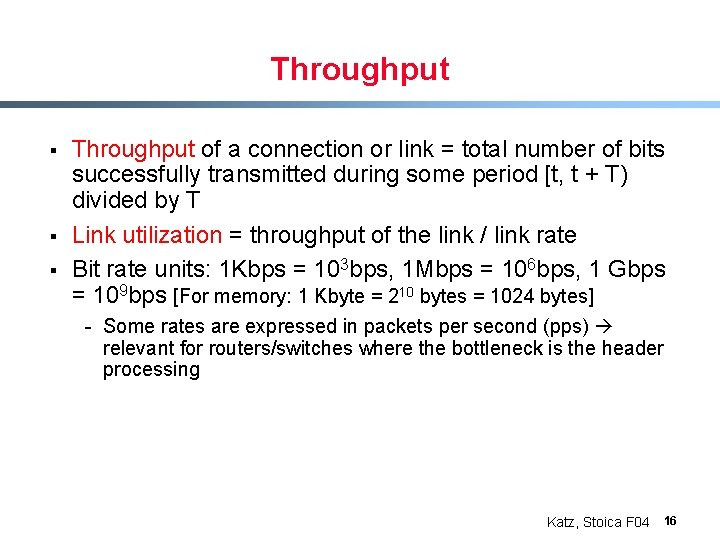Throughput § § § Throughput of a connection or link = total number of bits successfully transmitted during some period [t, t + T) divided by T Link utilization = throughput of the link / link rate Bit rate units: 1 Kbps = 103 bps, 1 Mbps = 106 bps, 1 Gbps = 109 bps [For memory: 1 Kbyte = 210 bytes = 1024 bytes] - Some rates are expressed in packets per second (pps) relevant for routers/switches where the bottleneck is the header processing Katz, Stoica F 04 16Example: Windows Based Flow Control § Connection: Source - Send W bits (window size) - Wait for ACKs - Repeat RTT § Assume the round-triptime is RTT seconds Throughput = W/RTT bps § Numerical example: RTT § - W = 64 Kbytes - RTT = 200 ms - Throughput = W/T = 2. 6 Mbps Destination time Katz, Stoica F 04 17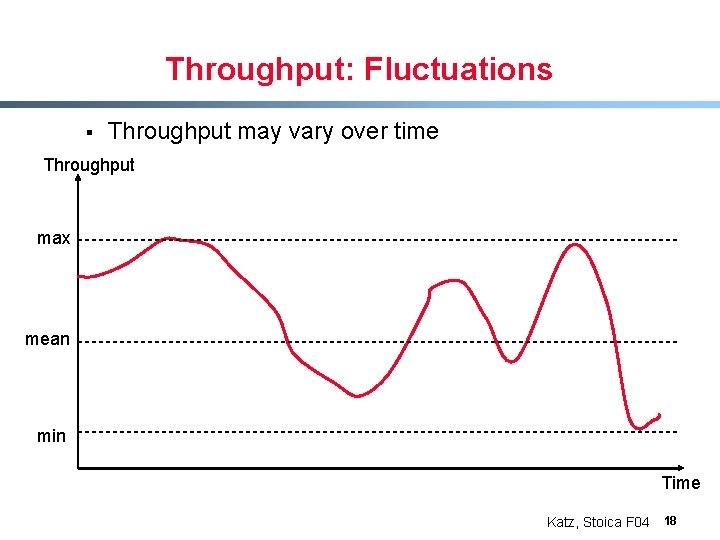Throughput: Fluctuations § Throughput may vary over time Throughput max mean min Time Katz, Stoica F 04 18Delay § Delay (Latency) of bit (packet, file) from A to B - The time required for bit (packet, file) to go from A to B § Jitter - Variability in delay § Round-Trip Time (RTT) - Two-way delay from sender to receiver and back § Bandwidth-Delay product - Product of bandwidth and delay “storage” capacity of network Katz, Stoica F 04 19Delay: Illustration 1 1 Sender Latest bit seen by time t 2 Receiver at point 2 at point 1 Delay time Katz, Stoica F 04 20Delay: Illustration 2 1 Sender 2 Receiver Packet arrival times at 1 1 2 Delay Packet arrival times at 2 Katz, Stoica F 04 21Little’s Theorem § § § Assume a system (e. g. , a queue) at which packets arrive at rate a(t) Let d(i) be the delay of packet i , i. e. , time packet i spends in the system What is the average number of packets in the system? d(i) = delay of packet i a(t) – arrival rate § system Intuition: - Assume arrival rate is a = 1 packet per second and the delay of each packet is s = 5 seconds - What is the average number of packets in the system? Katz, Stoica F 04 22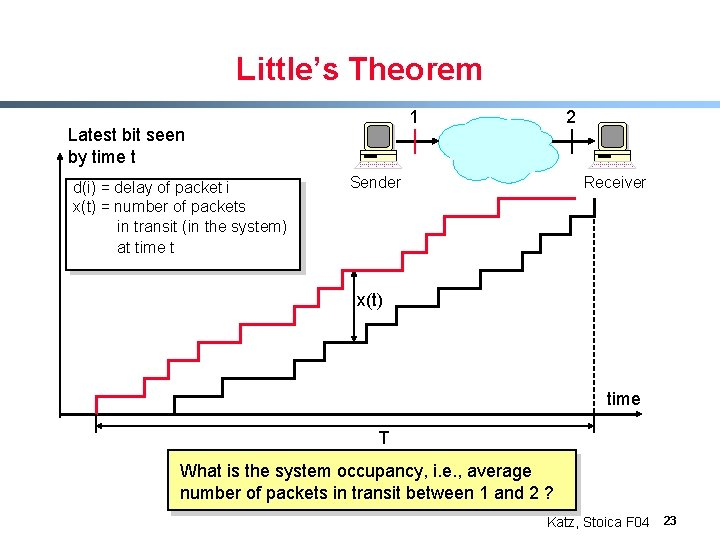Little’s Theorem 1 Latest bit seen by time t d(i) = delay of packet i x(t) = number of packets in transit (in the system) at time t 2 Sender Receiver x(t) time T What is the system occupancy, i. e. , average number of packets in transit between 1 and 2 ? Katz, Stoica F 04 23Little’s Theorem 1 Latest bit seen by time t d(i) = delay of packet i x(t) = number of packets in transit (in the system) at time t Sender 2 Receiver x(t) S= area time T Average occupancy = S/T Katz, Stoica F 04 24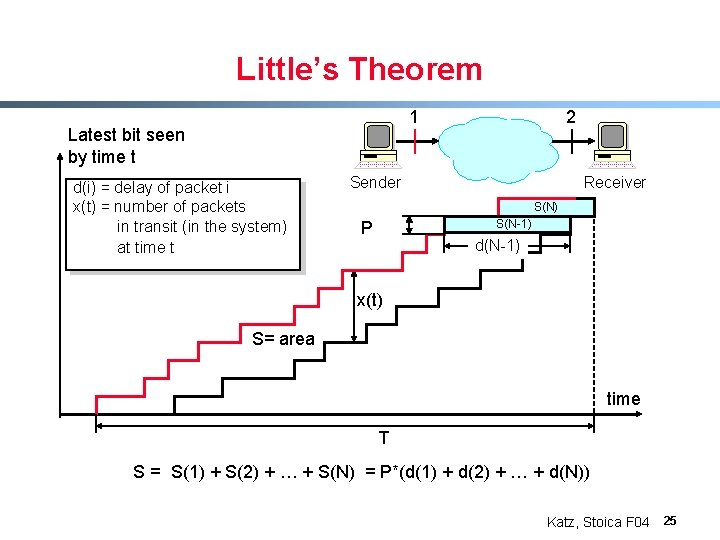Little’s Theorem 1 Latest bit seen by time t d(i) = delay of packet i x(t) = number of packets in transit (in the system) at time t 2 Sender Receiver S(N) S(N-1) P d(N-1) x(t) S= area time T S = S(1) + S(2) + … + S(N) = P*(d(1) + d(2) + … + d(N)) Katz, Stoica F 04 25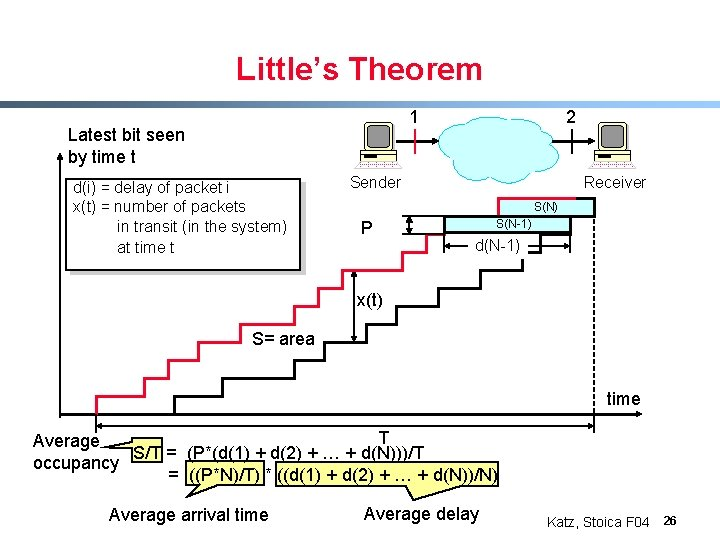Little’s Theorem 1 Latest bit seen by time t d(i) = delay of packet i x(t) = number of packets in transit (in the system) at time t 2 Sender Receiver S(N) P S(N-1) d(N-1) x(t) S= area time T Average S/T = (P*(d(1) + d(2) + … + d(N)))/T occupancy = ((P*N)/T) * ((d(1) + d(2) + … + d(N))/N) Average arrival time Average delay Katz, Stoica F 04 26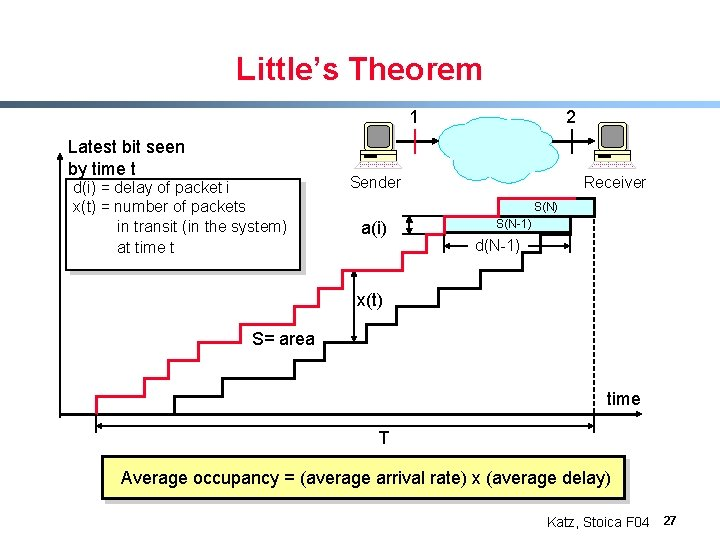Little’s Theorem 1 Latest bit seen by time t d(i) = delay of packet i x(t) = number of packets in transit (in the system) at time t 2 Sender Receiver S(N) a(i) S(N-1) d(N-1) x(t) S= area time T Average occupancy = (average arrival rate) x (average delay) Katz, Stoica F 04 27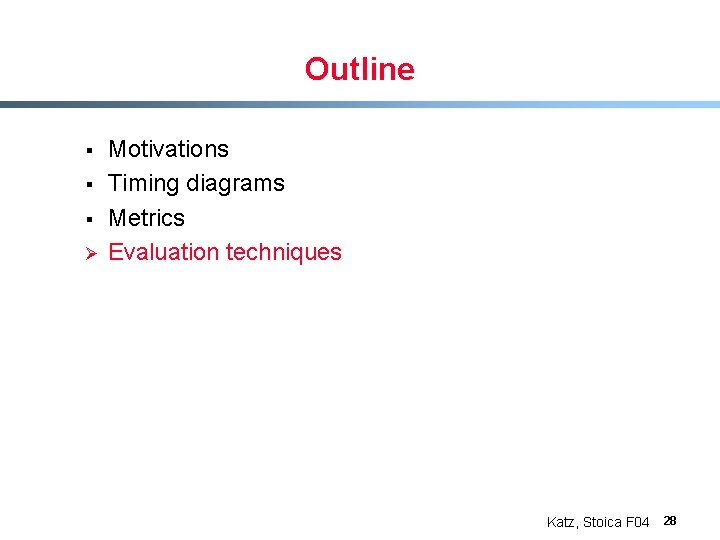Outline § § § Ø Motivations Timing diagrams Metrics Evaluation techniques Katz, Stoica F 04 28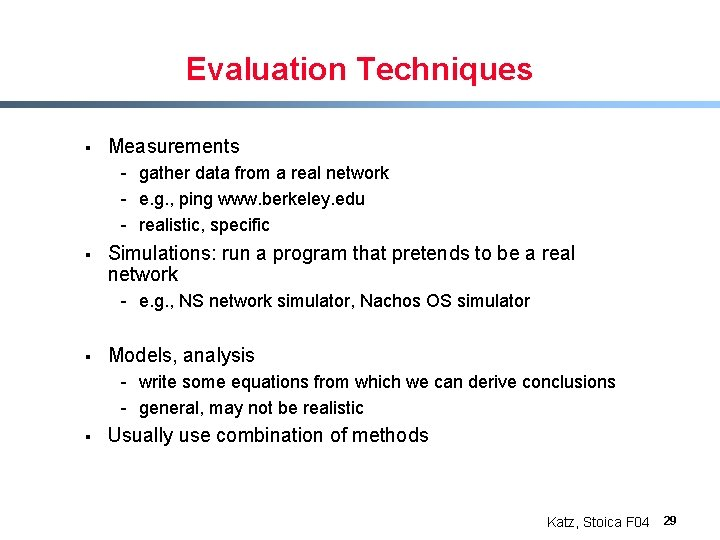Evaluation Techniques § Measurements - gather data from a real network - e. g. , ping www. berkeley. edu - realistic, specific § Simulations: run a program that pretends to be a real network - e. g. , NS network simulator, Nachos OS simulator § Models, analysis - write some equations from which we can derive conclusions - general, may not be realistic § Usually use combination of methods Katz, Stoica F 04 29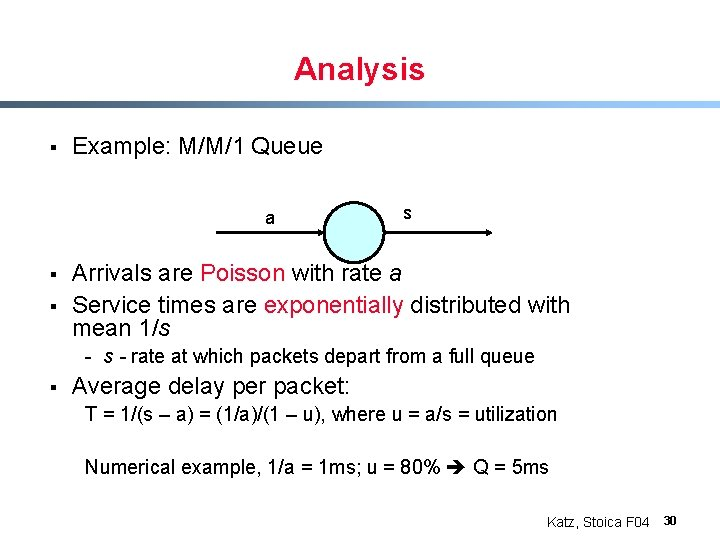Analysis § Example: M/M/1 Queue a § § s Arrivals are Poisson with rate a Service times are exponentially distributed with mean 1/s - rate at which packets depart from a full queue § Average delay per packet: T = 1/(s – a) = (1/a)/(1 – u), where u = a/s = utilization Numerical example, 1/a = 1 ms; u = 80% Q = 5 ms Katz, Stoica F 04 30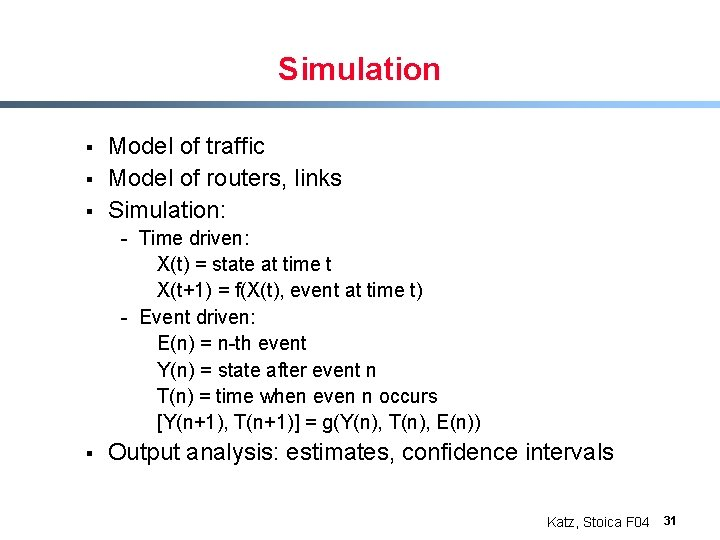Simulation § § § Model of traffic Model of routers, links Simulation: - Time driven: X(t) = state at time t X(t+1) = f(X(t), event at time t) - Event driven: E(n) = n-th event Y(n) = state after event n T(n) = time when even n occurs [Y(n+1), T(n+1)] = g(Y(n), T(n), E(n)) § Output analysis: estimates, confidence intervals Katz, Stoica F 04 31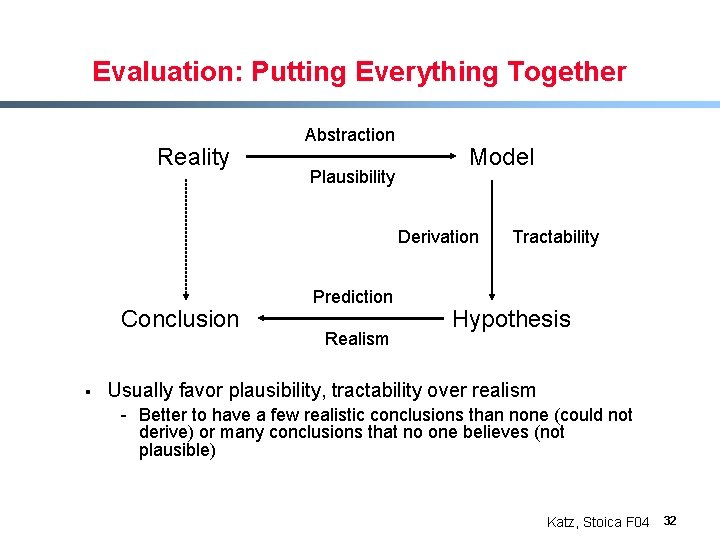Evaluation: Putting Everything Together Reality Abstraction Plausibility Model Derivation Conclusion § Prediction Realism Tractability Hypothesis Usually favor plausibility, tractability over realism - Better to have a few realistic conclusions than none (could not derive) or many conclusions that no one believes (not plausible) Katz, Stoica F 04 32